C Program to Interchange Numbers

In this tutorial you will learn about the C Program to Interchange Numbers and its application with practical example.

C Program to Interchange Numbers

In this tutorial, we will learn to create a C program that will Interchange Numbers in C programming.

Prerequisites

Before starting with this tutorial we assume that you are best aware of the following C programming topics:

• Operators in C Programming.
• Basic Input and Output function in C Programming.
• Basic C programming.
• While loop in C programming.

Program to Interchange Numbers:-

As we all know the c is a very powerful language. With the help of c programming language, we can make many programs. We can perform many input-output operations using c programming. In today’s tutorial, we take the input numbers from the user. Then we will Interchange Numbers with the help of the c programming language.

With the help of this program, we can Interchange Numbers.

Output:-In the above program, we have first initialized the required variable.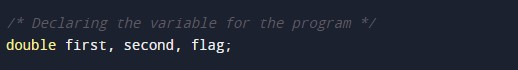• first= it will hold the double value.
• second = it will hold the double value.
• flag = it will hold the double value.

Taking the input number 1.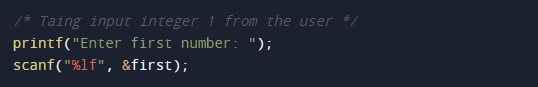Taking the input number 2.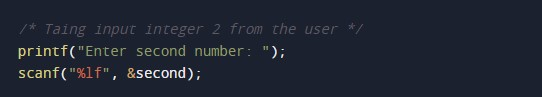Swapping the numbers.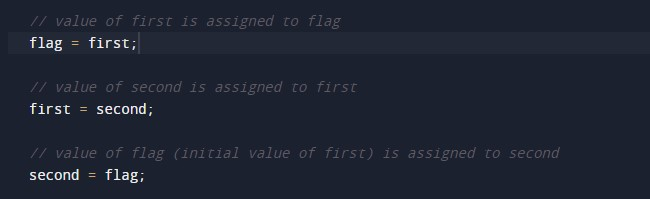Printing the output numbers.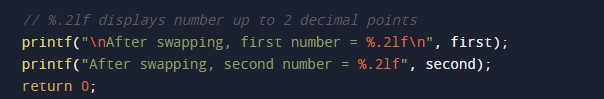In this tutorial we have learn about the C Program to Interchange Numbers and its application with practical example. I hope you will like this tutorial.